### Volt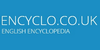The unit of electrical potential. (Italian physicist, Volta, 1745-1827).
Found on http://www.encyclo.co.uk/visitor-contributions.php

### volt[n] - a unit of potential equal to the potential difference between two points on a conductor carrying a current of 1 ampere when the power dissipated between the two points is 1 watt
Found on http://www.webdictionary.co.uk/definition.php?query=volt

### Volt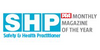Unit of potential difference.
Found on http://www.encyclo.co.uk/local/20474

### Volt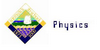The volt is the SI unit of voltage. In fact 1 V = 1 JC-1 (i.e. a charge of one coulomb will gain or lose one joule of potential energy when moved through a potential difference of one volt)
Found on http://www.users.zetnet.co.uk/computing/MainPage/SecDepts/Physics/Resources

### Volt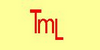A volt (after the physicist, Count Allesandro Volta) is the amount of elecromotive force required to make one ampere of current flow through one ohm of resistance.

### VoltThe volt is the SI unit of electromotive force. One volt produces a current of 1 amperethrough a resistance of 1 ohm. Electromotive force is the differenceof potential produced by an electrical source to drive a current through an externalelectrical circuit.Conversions1 volt=1.0x108 abvolts1 volt=1 J C-11 volt=0.003333 statvol...
Found on http://www.diracdelta.co.uk/science/source/v/o/volt/source.html

### volt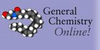(V) The SI unit of electrical potential. One volt equals one joule per coulomb.
Found on http://antoine.frostburg.edu/chem/senese/101/glossary/v.shtml

### volt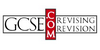The unit of electromotive force* or potential difference*.
Found on http://www.gcse.com/glos.htm

### Volt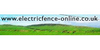Unit of electrical pressure which causes current to flow. Voltage is a measure of the rate at which electricity is moving. Calculation: Voltage = Current (Amps) x Resistance (Ohms). One volt drives a current of one amp through a resistance of one ohm. Current in a wire is driven by voltage in roughly the same way that water in your pipes is driven ...
Found on http://www.electricfence-online.co.uk/ishop/1047/shopscr21.html

### volt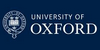The standard unit of potential difference or electromotive force, named after Alessandro Volta. One volt is the potential difference across a conductor when a current of one ampere dissipates one watt of power. The volt is the standard unit of voltage: the force, or pressure, of electricity.
Found on http://www.mhs.ox.ac.uk/marconi/collection/glossary.php

### Volt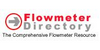The (electrical) potential difference between two points in a circuit. The fundamental unit is derived as work per unit charge-(V = W/Q). One volt is the potential difference required to move one coulomb of charge between two points in a circuit while using one joule of energy.
Found on http://www.flowmeterdirectory.com/flowmeter_technical_glossary/flowmeter_te

### VoltVolt noun [ French volte ; confer Italian volta . See Vault .] 1. (Man.) A circular tread; a gait by which a horse going sideways round a center makes two concentric tracks. 2. (Fencing) A sudden movement to avoid a thrust.
Found on http://www.encyclo.co.uk/webster/V/35

### volt<unit> Unit of electrical potential. ... (11 Jan 1998) ...
Found on http://www.encyclo.co.uk/local/20973

### voltV noun a unit of potential equal to the potential difference between two points on a conductor carrying a current of 1 ampere when the power dissipated between the two points is 1 watt; equivalent to the potential difference across a resistance of 1 ohm when 1...
Found on https://www.encyclo.co.uk/local/20974

### volt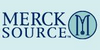(V) (vōlt) the SI unit of electric potential or electromotive force, equal to 1 watt per ampere, or 1 joule per coulomb. electron volt(eV) a unit of energy equal to the energy acquired by an electron when accelerated through a potential differ...
Found on http://www.encyclo.co.uk/local/21001

### Volt• (n.) The unit of electro-motive force; -- defined by the International Electrical Congress in 1893 and by United States Statute as, that electro-motive force which steadily applied to a conductor whose resistance is one ohm will produce a current of one ampere. It is practically equivalent to / the electro-motive force of a standard Clark`s ...
Found on http://thinkexist.com/dictionary/meaning/volt/

### voltunit of electrical potential, potential difference and electromotive force in the metre–kilogram–second system (SI); it is equal to the difference ... [10 related articles]
Found on http://www.britannica.com/eb/a-z/v/34

### Volt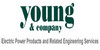A unit of electromotive force. The electrical potential needed to produce one ampere of current with a resistance of one ohm.
Found on http://www.youngco.com/young2.asp?ID=4&Type=3

### Volt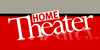The unit of electrical potential, or difference in electrical pressure, expressing the difference between two electrical charges.
Found on http://www.encyclo.co.uk/local/21058

### volt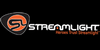Unit of electrical potential. The potential difference between two points in an electrical system is called the voltage between those points.
Found on http://www.streamlight.com/education/glossary.xhtml

### Volt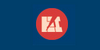A unit of electrical force equal to that amount of electromotive force that will cause a steady current of one ampere to flow through a resistance of one ohm.
Found on http://www.electromn.com/glossary/v.htm

### voltThe SI unit of electromotive force or electrical potential difference. The volt is defined as the potential difference across a conductor when a current of one ampere dissipates one watt of power. It is named after the Italian physicist Alessandro Volta.
Found on http://www.daviddarling.info/encyclopedia/V/volt.html

### voltA unit of electrical force, or 'push,' given to the electrons in an electric circuit. One volt is the electromotive force that will cause a steady current of one ampere to flow through a resistance of one ohm. Related category • UNITS
Found on http://www.daviddarling.info/encyclopedia/V/AE_volt.html

### volt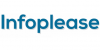volt [for Alessandro Volta], abbr. V, unit of electric potential and electromotive force. It is defined as the difference of electric potential existing across the ends of a conductor carrying a constant current of 1 ampere when the power dissipated is 1 watt. The kilovolt (1,000 V), the millivolt (...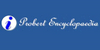The volt is the unit of electromotive force. It was named after Alessandro Volta.